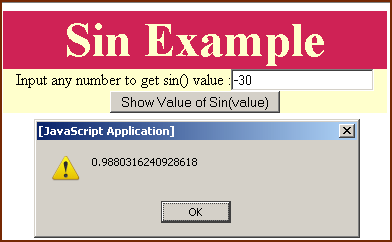Tutorials

JavaScript Math.sin() method

JavaScript's Math.sin() method returns mathematical sine of the number and it

JavaScript's Math.sin() method returns mathematical sine of the number and it

JavaScript Math.sin() method

JavaScript's Math.sin() method returns mathematical sine of the number and it returns value between -1 to 1.

Syntax:

 Math.sin( value );

where "value" is a numeric value and is required. To illustrate use of sin() method we are providing you a simple example.

Description of code:

In this example we have created an HTML page and in this page we have created an input box into which user can input the values for sin() method. We have also created a button labled "Show value of Sin(value)" which calls function callSin() as defined in the JavaScript.

 function callSin(){ var val= document.getElementById("txt").value; alert(Math.sin(val)); }

The very first line of function takes the input value into a variable "val". In the second line we have supplied this value into the method sin(). Alert message shows the sine value of "val". Here is the full description of code as follows :

 Sin method example
Sin Example
Input any number to get sin() value :

Output :

Output of the page would look like as given below :We can also pass a negative numeric to the sin() method as we have passed in the following :